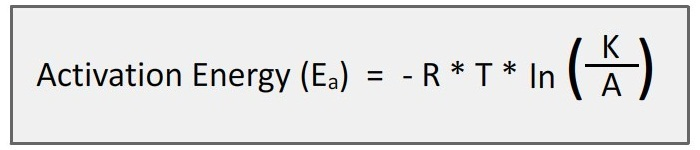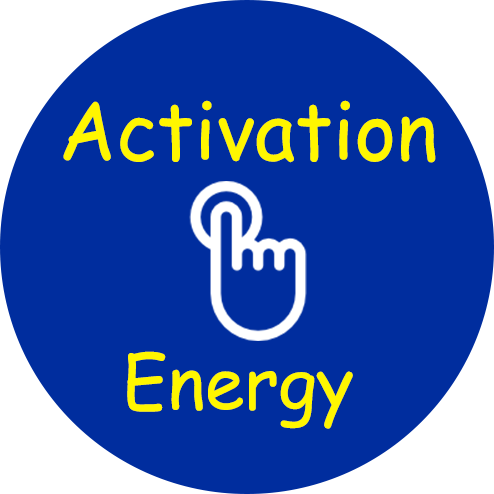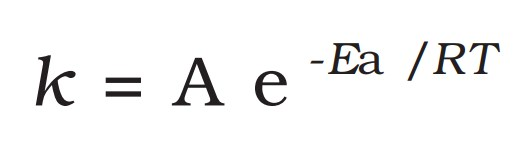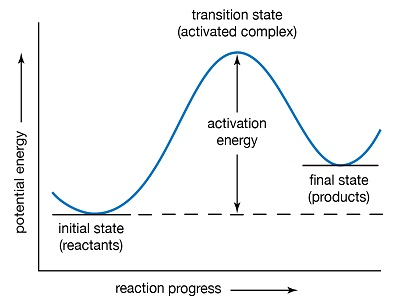# Activation Energy Calculator

Use our activation energy calculator a free online tool to calculate the activation energy which is required for the atoms to undergo the chemical reaction. The Activation Energy (Ea) is the energy level that the reactant molecules must overcome before a reaction can occur. Enter the values of temperature in kelvin, rate constant and frequency factor to calculate.Where, R is the Universal Gas Constant; (R) = 8.31447215 J K−1 mol−1

Ea is the activation energy of the reaction

K is the reaction rate coefficient. It is measured in 1/sec and dependent on temperature.

T is the temperature of the surroundings, expressed in Kelvins.

A is the pre-exponential factor (also called the frequency factor), expressed in 1/sec.### What is Activation Energy and Why it is Important ?

The temperature dependence of the rate of a chemical reaction can be accurately explained by Arrhenius equation.

#### Arrhenius EquationIt was first proposed by Dutch chemist, J.H. van’t Hoff but Swedish chemist, Arrhenius provided its physical justification and interpretation.

Here, A is the Arrhenius factor or the frequency factor. It is also called pre-exponential factor. It is a constant specific to a particular reaction.

R is gas constant and Ea is activation energy measured in joules/mole(J mol –1).

#### Activation Energy

The minimum extra amount of energy absorbed by reactant molecules so that their energy becomes equal to the threshold energy is called activation energy.

Activation energy = Threshold energy - Kinetic energy### Activated complex (or transition state)

Activated complex is the highest energy unstable intermediate between the reactants and products and gets decomposed immediately (having very short life), to give the products. In this state, bonds of reactant are not completely broken while the bonds of products are not completely formed.

Threshold energy (ET) The minimum amount of energy which the reactant must possess in order to convert into products is known as threshold energy.

Activation energy (Ea) The additional amount of energy, required by the reactant so that their energy becomes equal to the threshold value is known as activation energy.

Ea = ET – ER

Lower the activation energy, faster is the reaction.